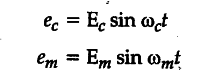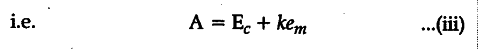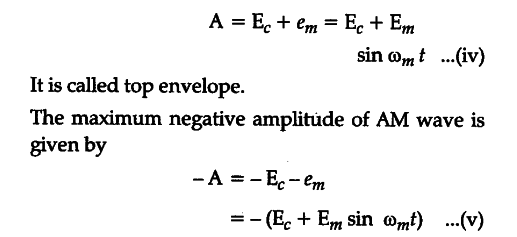# Distinguish between sinusoidal and pulse-shaped signals

a) Distinguish between sinusoidal and pulse-shaped signals.
(b) Explain, showing graphically, how a sinusoidal carrier wave is superimposed on a modulating signal to obtain the resultant amplitude modulated (AM) wave.

(a) In the process of; modulation, some specific characteristics of the carrier wave is varied in accordance with the information or message signal. The carrier wave may be :
(i) continuous (sinusoidal) wave, or
(ii) pulse, which is discontinuous
A continuous sinusoidal carrier wave can be expressed as

Three distinct characteristics of such a wave are amplitude (Eo), angular frequency (to) and phase angle . Any one of these three characteristics can be varied in accordance with the modulating baseband (AF) signal, giving rise to the respective amplitude modulation; frequency modulation and phase modulation.
Again, the significant characteristics of a pulse are pulse amplitude, pulse duration or pulse width and pulse position (representing the time of rise or fall of the pulse amplitude). Any one of these characteristics can be varied in accordance with the modulating baseband (AF) signal, giving rise to the respective, pulse amplitude modulation (PAM), pulse duration modulation (PDM) or pulse width modulation (PWM) and pulse position modulation (PPM).
(b) Amplitude Modulation : When a modulating AF wave is superimposed on a high frequency carrier wave in a manner that the frequency of modulated wave is same as that of the carrier wave, but its amplitude is made proportioned to the instantaneous amplitude of the audio frequency modulating voltage, the process is called amplitude modulation (AM).
Let the instantaneous carrier voltage (ec) and modulating voltage (em) be represented byThus, in amplitude moduation, amplitude A of modulated wave is made proportional to the instantaneous modulating voltagewhere k is a constant of proportionality.
In amplitude modulation, the proportionality constant k is made equal to unity. Therefore, maximum positive amplitude of AM wave is given by